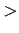Next: 4.2 MLN Syntax Up: 4 Syntax Previous: 4 Syntax

## 4.1 First-Order Logic

You can express an arbitrary first-order formula in an .mln file. The syntax for logical connectives is as follows: ! (not), ^ (and), v (or), => (implies), <=> (if and only if), FORALL/forall/Forall (universal quantification), and EXIST/exist/Exist (existential quantification). Operator precedence is as follows: notandorimpliesif and only ifforall = exists. Operators with the same precedence are evaluated left to right. You can use parentheses to enforce a different precedence, or to make precedence explicit (e.g., (A=>B)=>C as opposed to A=>(B=>C)). Universal quantifiers at the outermost level can be omitted, i.e., free variables are interpreted as universally quantified at the outermost level. Quantifiers can be applied to more than one variable at once (e.g., forall x,y). The infix equality sign (e.g., x = y) can be used as a shorthand for the equality predicate (e.g., equals(x,y)).

Marc Sumner 2010-01-22